### Reports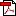473 kb
Date : 01 Jul 2021
Annex 2: Methodologies

2.1 Scheduled Commercial Banks

Banking stability map and indicator

The banking stability map and indicator present an overall assessment of changes in underlying conditions and risk factors that have a bearing on the stability of the banking sector during a period. The five composite indices used in the banking stability map and indicator represent the five dimensions of soundness, asset-quality, profitability, liquidity and efficiency. The ratios used for constructing each composite index are given in Table 1.

 Table 1: Ratios used for constructing the banking stability map and indicator Dimension Ratios Soundness CRAR # Tier-I Capital to Tier-II Capital # Leverage Ratio as Total Assets to Capital and Reserves Asset-Quality Net NPAs to Total Advances Gross NPAs to Total Advances Sub-Standard Advances to Gross NPAs # Restructured Standard Advances to Standard Advances Profitability Return on Assets # Net Interest Margin # Growth in Profit # Liquidity Liquid Assets to Total Assets # Customer Deposits to Total Assets # Non-Bank Advances to Customer-Deposits Deposits maturing within 1-year to Total Deposits Efficiency Cost to Income Business (Credit + Deposits) to Staff Expenses # Staff Expenses to Total Expenses Note: # Negatively related to risk.

Each composite index, representing a dimension of bank functioning, takes values between zero and one. Each index is a relative measure during the sample period used for its construction, where a higher value means the risk in that dimension is high. Therefore, an increase in the value of the index in any particular dimension indicates an increase in risk in that dimension for that period as compared to other periods. Each index is normalised for the sample period using the following formula: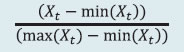Where, Xt is the value of the ratio at time t. A composite index of each dimension is calculated as a weighted average of normalised ratios used for that dimension where the weights are based on the marks assigned for assessment for the CAMELS rating. The banking stability indicator is constructed as a simple average of these five composite indices.

Macro stress testing

Macro stress test for credit risk ascertains the resilience of banks against macroeconomic shocks. It assesses the impact of macroeconomic shocks on GNPA ratio of banks (at system level and at major bank-group level) and finally on their capital adequacy (bank-by-bank and system level for a sample of 46 banks).

Impact of GNPA ratio

Here, the slippage ratio (SR)1 is modelled as a function of macroeconomic variables, using various econometric models that relate the select banking system aggregates to macroeconomic variables. The system-level and bank group-level slippage ratios are modelled using (i) multivariate regression; (ii) VAR and (iii) quantile regression. The banking system aggregates include current and lagged values of slippage ratio, while macroeconomic variables include gross domestic product (GDP), weighted average lending rate (WALR), CPI (combined) inflation, exports-to-GDP ratio, annualized current account balance-to-GDP ratio and annualized combined gross fiscal deficit-to-GDP ratio.

While multivariate regression allows evaluating the impact of select macroeconomic variables on the banking system’s GNPA, the VAR model takes into account the feedback effect also. In these methods, the conditional mean of slippage ratio is estimated wherein it is assumed that the impact of macro-variables on credit quality will remain the same, irrespective of the level of the credit quality, which may not always be true. In order to relax this assumption, quantile regression was adopted, wherein conditional quantile is estimated instead of the conditional mean to deal with tail risks and to account for the non-linear impact of macroeconomic shocks.

The following econometric models are used to estimate the impact of macroeconomic shocks on the slippage ratio:

System level models

The system level GNPAs are projected using three different but complementary econometric models: multivariate regression, VAR and quantile regression. The final projection is derived by averaging the projections based on these three models.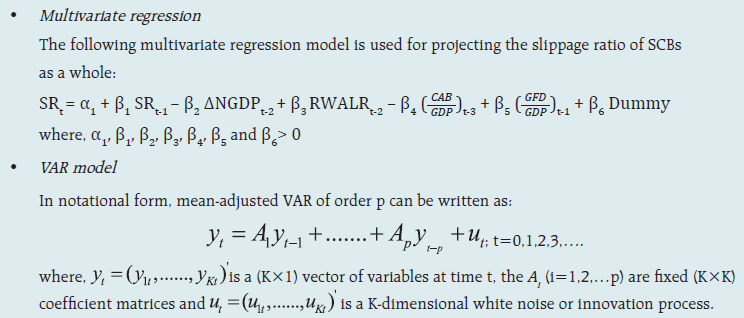The VAR model is estimated using slippage ratio, real WALR, nominal GDP growth, annualized current account balance-to-GDP ratio and annualized combined gross fiscal deficit-to-GDP ratio. The appropriate order of VAR selected based on minimum information criteria as well as other diagnostics is two. The impact of various macroeconomic shocks is determined using the impulse response function of the selected VAR.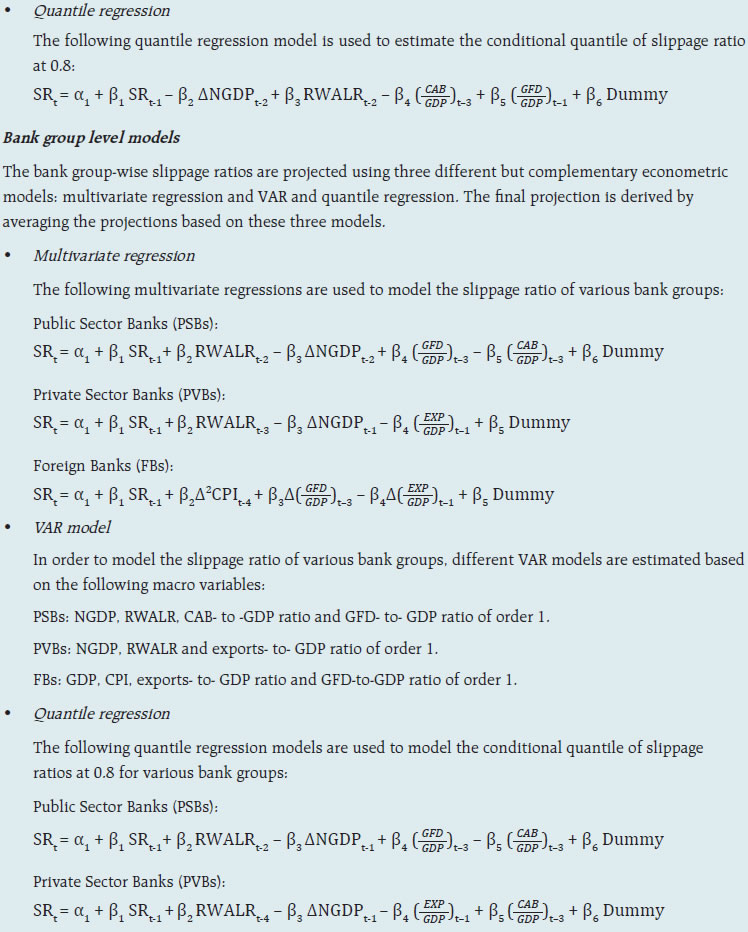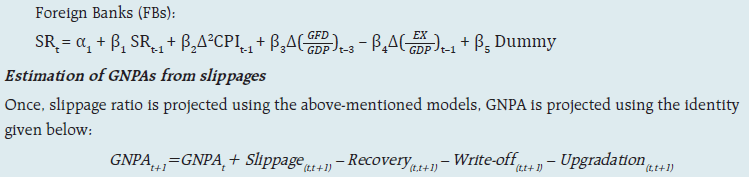Derivation of GNPAs from slippage ratios, which are projected using the above mentioned credit risk econometric models, are based on the following assumptions: credit growth of 5.7 per cent, 6.4 per cent, 7.2 per cent and 7.9 per cent respectively; recovery rates of 2.5 per cent, 2.5 per cent, 3.6 per cent and 3.4 per cent, respectively; write-off rates of 5.2 per cent, 4.3 per cent, 5.7 per cent and 8.2 per cent respectively; upgradation rates of 1.4 per cent, 1.1 per cent, 1.0 per cent and 1.3 per cent respectively during quarters ending June 2021, September 2021, December 2021 and March 2022.

Impact on capital adequacy

The impact of macro shocks on capital adequacy of banks is captured through the following steps;

i. The impact on future capital accumulation is captured through projection of profit under the assumed macro scenarios, assuming that only 25 per cent of profit after tax (PAT) (which is minimum regulatory requirements) goes into capital of banks.

ii. The requirement of additional capital in future are projected by estimating risk-weighted assets (RWAs) using internal rating based (IRB) formula.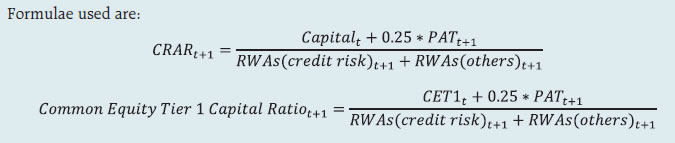where, PAT is projected using satellite models, elucidated in the subsequent section. RWAs (others), which is total RWAs minus RWAs of credit risk, is projected based on average growth rate observed in the past one year. RWAs (credit risk) is estimated using the IRB formula given below:

IRB Formula: Bank-wise RWA for credit risk is estimated using the following IRB formula;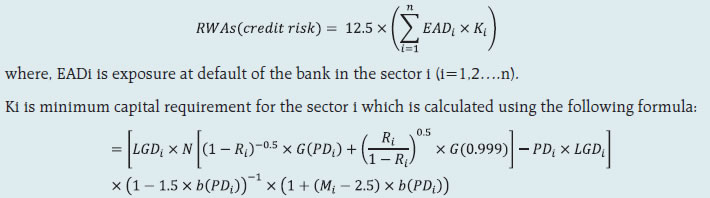where, LGDi is loss given default of the sector i, PDi is probability of default of the sector i, N(..) is cumulative distribution function of standard normal distribution, G(..) is inverse of cumulative distribution function of standard normal distribution, Mi is average maturity of loans of the sector (which is taken 2.5 for all the sector in this case), b(PDi) is smoothed maturity adjustment and Ri is correlation of the sector i with the general state of the economy. Calculation of both, b(PD) and R depend upon PD.

This IRB formula requires three major inputs, namely, sectoral PD, EAD and LGD. Here, sectoral PDs are proxied by annual slippage of the respective sectors using banking data. PD for a particular sector is taken as same (i.e. systemic shocks) for each of the 46 selected banks, whereas, EAD for a bank for a particular sector is total outstanding loan (net of NPAs) of the bank in that particular sector. Further, assumption on LGD was taken as follows; under the baseline scenario, LGD = 60 per cent (broadly as per the RBI guidelines on ‘Capital Adequacy - The IRB Approach to Calculate Capital Requirement for Credit Risk’), which increases to 65 per cent under medium macroeconomic risk scenario and 70 per cent under severe macroeconomic risk.

Selected sectors: The following 17 sectors/sub-sectors (and others) are selected for the stress test.

 Table 2: List of selected sectors/sub-sectors Sr. No. Sector/Sub-sector Sr. No. Sector/Sub-sector 1 Engineering 10 Basic Metal and Metal Products 2 Auto 11 Mining 3 Cement 12 Paper 4 Chemicals 13 Petroleum 5 Construction 14 Agriculture 6 Textiles 15 Retail-Housing 7 Food Processing 16 Retail-Others 8 Gems and Jewellery 17 Services 9 Infrastructure 18 Others

The stochastic relationship of sectoral annual slippage ratio (i.e. sectoral PDs) with macro variables is estimated using multivariate regression for each sector. Using these estimated regressions, sectoral PDs of each sector are projected for four quarters ahead under assumed baseline as well as two adverse scenarios, namely, medium stress and severe stress. The sectoral regression models are presented in the next section.

The bank-wise profit after tax (PAT) is projected using the following steps:

• Components of PAT (i.e. Net Interest Income(NII), Other Operating Income(OOI), Operating Expenses(OE) and Provisions & Write off) of each bank-group is projected under baseline and adverse scenarios, using the method explained in the subsequent section.

• Share of components of PAT of each bank (except income tax) in their respective bank-group is calculated.

• Each component of PAT (except income tax) of each bank is projected from the projected value of the component of PAT of respective bank-group and applying that bank’s share in the particular component of PAT.

• Finally, bank-wise PAT was projected by appropriately adding or subtracting their components estimated in the previous step and using income tax rate at 35 per cent.

Using these formulae, assumptions and inputs, impact of assumed macro scenarios on the capital adequacy of each bank is estimated and future change in capital adequacy under baseline from the latest observed data and change in the capital adequacy of banks from baseline to adverse macro shocks are calculated. Finally, these changes are appropriately applied on the latest observed capital adequacy (under Standardised Approach) of the bank.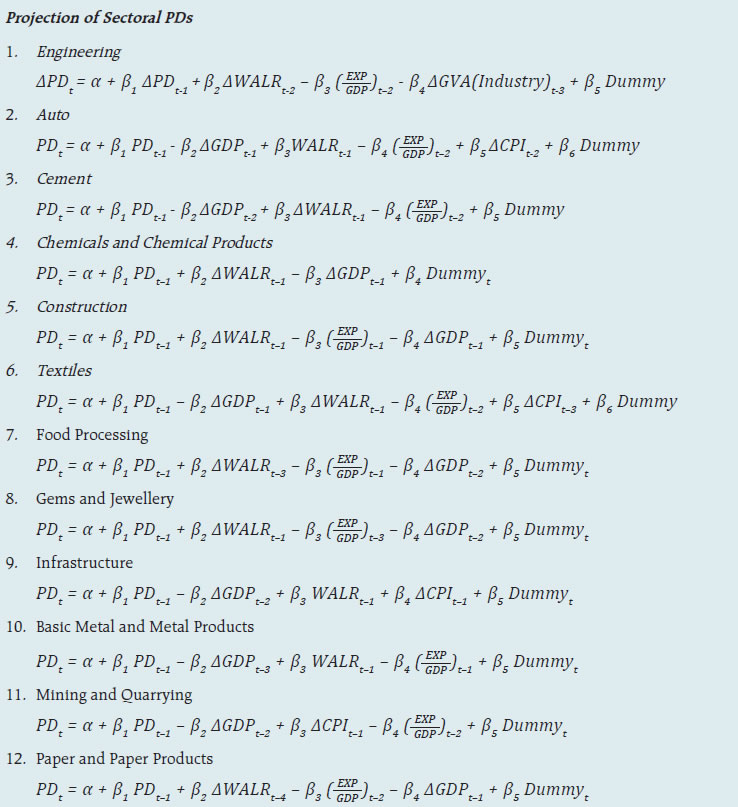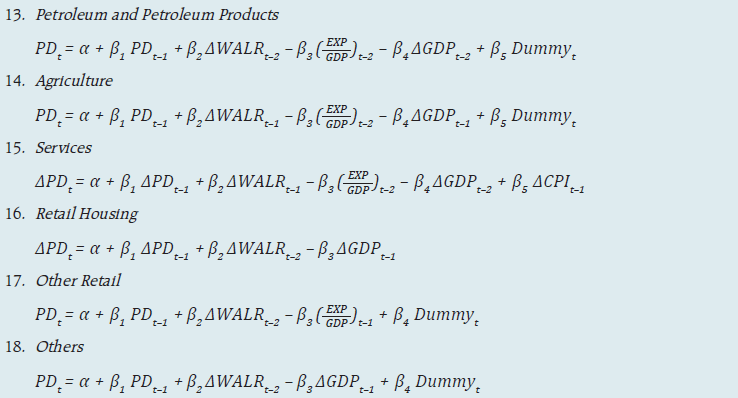Projection of bank-group wise PAT

The various components of PAT of major bank-groups (namely, PSBs, PVBs and FBs), such as, NII, OOI, OE and Provisions & Writeoff are projected using different time series econometric models (as given below). Finally, PAT is estimated using the following identity: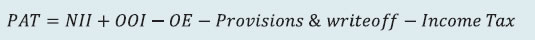where, NII is net interest income, OOI is other operating income and OE is operating expenses.

Net Interest Income (NII): NII is the difference between interest income and interest expense and is projected using the following regression model: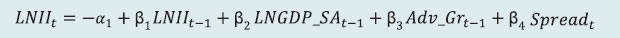LNII is log of NII. LNGDP_SA is seasonally adjusted log of nominal GDP. Adv_Gr is the y-o-y growth rate of loans and advances. Spread is the difference between average interest rate earned by interest earning assets and average interest paid on interest bearing liabilities.

Other Operating Income (OOI): Log of OOI (LOOI) of SCBs is projected using the following regression model: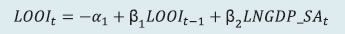Operating Expense (OE): OE of SCBs is projected using an Autoregressive Moving Average (ARMA) model.

Provisions (including write-off): The required provisioning is projected using the following regression: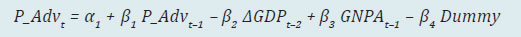P_Adv is provisions to total advances ratio. ΔGDP is the y-o-y growth rate of real GDP. GNPA is gross non-performing assets to total advances ratio.

Income Tax: The applicable income tax is taken as 35 per cent of profit before tax, which is based on the past trend of ratio of income tax to profit before tax.

Single factor sensitivity analysis – Stress testing

As a part of quarterly surveillance, stress tests are conducted covering credit risk, interest rate risk, liquidity risk etc. and the resilience of commercial banks in response to these shocks is studied. The analysis is done on individual SCBs as well as on the system level.

Credit risk (includes concentration risk)

To ascertain the resilience of banks, the credit portfolio was given a shock by increasing GNPA ratio for the entire portfolio. For testing the credit concentration risk, default of the top individual borrower(s) and the largest group borrower(s) was assumed. The analysis was carried out both at the aggregate level as well as at the individual bank level. The assumed increase in GNPAs was distributed across sub-standard, doubtful and loss categories in the same proportion as prevailing in the existing stock of NPAs. However, for credit concentration risk (exposure based) the additional GNPAs under the assumed shocks were considered to fall into sub-standard category only and for credit concentration risk (based on stressed advances), stressed advances were considered to fall into loss category. The provisioning requirements were taken as 25 per cent, 75 per cent and 100 per cent for sub-standard, doubtful and loss advances respectively. These norms were applied on additional GNPAs calculated under a stress scenario. As a result of the assumed increase in GNPAs, loss of income on the additional GNPAs for one quarter was also included in total losses, in addition to the incremental provisioning requirements. The estimated provisioning requirements so derived were deducted from banks’ capital and stressed capital adequacy ratios were computed.

Sectoral Risk

To ascertain the Sectoral credit risk of individual banks, the credit portfolios of particular sector was given a shock by increasing GNPA ratio for the sector. The analysis was carried out both at the aggregate level as well as at the individual bank level. Sector specific shocks based on standard deviation(SD) of GNPA ratios of a sector are used to study the impact on individual banks. The additional GNPAs under the assumed shocks were considered to fall into sub-standard category only. As a result of the assumed increase in GNPAs, loss of income on the additional GNPAs for one quarter was also included in total losses, in addition to the incremental provisioning requirements. The estimated provisioning requirements so derived were deducted from banks’ capital and stressed capital adequacy ratios were computed.

Interest rate risk

Under assumed shocks of the shifting of the INR yield curve, there could be losses on account of the fall in value of the portfolio or decline in income. These estimated losses were reduced from the banks’ capital to arrive at stressed CRAR.

For interest rate risk in the trading portfolio (HFT + AFS), a duration analysis approach was considered for computing the valuation impact (portfolio losses). The portfolio losses on these investments were calculated for each time bucket based on the applied shocks. The resultant losses/gains were used to derive the impacted CRAR.

Equity price risk

Under the equity price risk, impact of a shock of a fall in the equity price index, by certain percentage points, on profit and bank capital were examined. The fall in value of the portfolio or income losses due to change in equity prices are accounted for the total loss of the banks because of the assumed shock. The estimated total losses so derived were reduced from the banks’ capital.

Liquidity risk

The aim of the liquidity stress tests is to assess the ability of a bank to withstand unexpected liquidity drain without taking recourse to any outside liquidity support. Various scenarios depict different proportions (depending on the type of deposits) of unexpected deposit withdrawals on account of sudden loss of depositors’ confidence along with a demand for unutilised portion of sanctioned/committed/guaranteed credit lines (taking into account the undrawn working capital sanctioned limit, undrawn committed lines of credit and letters of credit and guarantees). The stress tests were carried out to assess banks’ ability to fulfil the additional and sudden demand for credit with the help of their liquid assets alone.

Assumptions used in the liquidity stress tests are given below:

• It is assumed that banks will meet stressed withdrawal of deposits or additional demand for credit through sale of liquid assets only.

• The sale of investments is done with a haircut of 10 per cent on their market value.

• The stress test is done under a ‘static’ mode.

Bottom-up Stress testing: Select banks

Bottom-up sensitivity analysis was performed by 18 select scheduled commercial banks. A set of common scenarios and shock sizes were provided to the select banks. The tests were conducted using March 2021 data. Banks used their own methodologies for calculating losses in each case.

Bottom-up stress testing: Derivatives portfolios of select banks

The stress testing exercise focused on the derivatives portfolios of a representative sample set of top 20 banks in terms of notional value of the derivatives portfolios. Each bank in the sample was asked to assess the impact of stress conditions on their respective derivatives portfolios.

In case of domestic banks, the derivatives portfolio of both domestic and overseas operations was included. In case of foreign banks, only the domestic (Indian) position was considered for the exercise. For derivatives trade where hedge effectiveness was established it was exempted from the stress tests, while all other trades were included.

The stress scenarios incorporated four sensitivity tests consisting of the spot USD/INR rate and domestic interest rates as parameters.

 Table 3: Shocks for stress testing of derivatives portfolio Domestic interest rates Shock 1 Overnight +2.5 percentage points Up to 1yr +1.5 percentage points Above 1yr +1.0 percentage points Domestic interest rates Shock 2 Overnight -2.5 percentage points Up to 1yr -1.5 percentage points Above 1yr -1.0 percentage points Exchange rates Shock 3 USD/INR +20 per cent Exchange rates Shock 4 USD/INR -20 per cent

2.2 Scheduled Primary (urban) Co-operative Banks

Single factor sensitivity analysis – Stress testing

Credit risk

Stress tests on credit risk were conducted on SUCBs. The tests were based on a single factor sensitivity analysis. The impact on CRAR was studied under following four different scenarios, using the historical standard deviations (SD).

• Scenario A: 1 SD shock to GNPA (incremental NPAs classified as sub-standard advances),

• Scenario B: 2 SD shock to GNPA (incremental NPAs classified as sub-standard advances),

• Scenario C: 1 SD shock to GNPA (incremental NPAs classified as loss advances),

• Scenario D: 2 SD shock to GNPA (incremental NPAs classified as loss advances).

Liquidity risk

A liquidity stress test based on a cash flow basis in the 1-28 days time bucket was also conducted, where mismatch [negative gap (cash inflow less cash outflow)] exceeding 20 per cent of outflow was considered stressful.

• Scenario A: Cash outflows in the 1-28 days time-bucket goes up by 50 per cent (no change in cash inflows).

• Scenario B: Cash outflows in the 1-28 days time-bucket goes up by 100 per cent (no change in cash inflows).

2.3 Non-banking Financial Companies

Single factor sensitivity analysis – Stress testing

Credit risk

Credit portfolio of NBFCs at individual level and system level was applied a shock by increasing the GNPA ratio by 1-SD and 2-SD under medium and high-risk scenarios. Baseline scenario was presented based on capital adequacy position of NBFCs reported as on March 2021. Credit exposure and RWA were assumed to grow at 75 per cent of compound annual growth rate (CAGR) over past three years. Additional NPAs were added to sub-standard advances and existing GNPA was distributed based on ageing impact as per the extant regulations. Provisioning requirements were applied at 10% for substandard advances, at the existing proportion as on March 2021 for doubtful advances and at 100% for loss advances as per the regulatory requirements. Additional provision requirements and income loss due to increase in GNPA were deducted from the earnings before provisions and taxes (EBPT) for FY2020-21 to calculate new profit before tax (PBT). Tax rate of 22 per cent was applied to calculate profit after tax (PAT) and complete PAT was accrued to existing capital with no dividend payment assumption. Based on the new capital and RWA, new capital to risk weighted assets ratio (CRAR) for individual NBFCs and entire sector were calculated for the assumed scenarios.

2.4 Interconnectedness – Network analysis

Matrix algebra is at the core of the network analysis, which uses the bilateral exposures between entities in the financial sector. Each institution’s lendings to and borrowings from all other institutions in the system are plotted in a square matrix and are then mapped in a network graph. The network model uses various statistical measures to gauge the level of interconnectedness in the system. Some of the important measures are given below: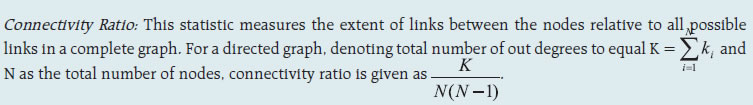Cluster coefficient: Clustering in networks measures how interconnected each node is. Specifically, there should be an increased probability that two of a node’s neighbours (banks’ counterparties in case of a financial network) are neighbours to each other also. A high clustering coefficient for the network corresponds with high local interconnectedness prevailing in the system. For each bank with ki neighbours the total number of all possible directed links between them is given by ki (ki-1). Let Ei denote the actual number of links between agent i’s ki neighbours, viz. those of i’s ki neighbours who are also neighbours. The clustering coefficient Ci for bank i is given by the identity: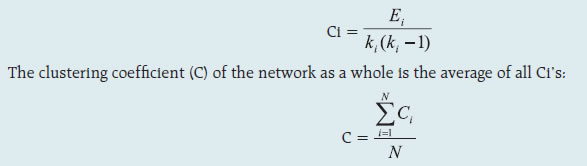Tiered network structures: Typically, financial networks tend to exhibit a tiered structure. A tiered structure is one where different institutions have different degrees or levels of connectivity with others in the network. In the present analysis, the most connected banks are in the innermost core. Banks are then placed in the mid-core, outer core and the periphery (the respective concentric circles around the centre in the diagrams), based on their level of relative connectivity. The range of connectivity of the banks is defined as a ratio of each bank’s in-degree and out-degree divided by that of the most connected bank. Banks that are ranked in the top 10 percentile of this ratio constitute the inner core. This is followed by a mid-core of banks ranked between 90 and 70 percentile and a 3rd tier of banks ranked between the 40 and 70 percentile. Banks with a connectivity ratio of less than 40 per cent are categorised as the periphery.

Colour code of the network chart: The blue balls and the red balls represent net lender and net borrower banks respectively in the network chart. The colour coding of the links in the tiered network diagram represents the borrowing from different tiers in the network (for example, the green links represent borrowings from the banks in the inner core).

Solvency contagion analysis

The contagion analysis is in nature of stress test where the gross loss to the banking system owing to a domino effect of one or more banks failing is ascertained. We follow the round by round or sequential algorithm for simulating contagion that is now well known from Furfine (2003). Starting with a trigger bank i that fails at time 0, we denote the set of banks that go into distress at each round or iteration by Dq, q= 1,2, …For this analysis, a bank is considered to be in distress when its Tier-I CRAR goes below 7 per cent. The net receivables have been considered as loss for the receiving bank.

Liquidity contagion analysis

While the solvency contagion analysis assesses potential loss to the system owing to failure of a net borrower, liquidity contagion estimates potential loss to the system due to the failure of a net lender. The analysis is conducted on gross exposures between banks. The exposures include fund based and derivatives ones. The basic assumption for the analysis is that a bank will initially dip into its liquidity reserves or buffers to tide over a liquidity stress caused by the failure of a large net lender. The items considered under liquidity reserves are: (a) excess CRR balance; (b) excess SLR balance; and (c) 18 per cent of NDTL. If a bank is able to meet the stress with liquidity buffers alone, then there is no further contagion.

However, if the liquidity buffers alone are not sufficient, then a bank will call in all loans that are ‘callable’, resulting in a contagion. For the analysis only short-term assets like money lent in the call market and other very short-term loans are taken as callable. Following this, a bank may survive or may be liquidated. In this case there might be instances where a bank may survive by calling in loans, but in turn might propagate a further contagion causing other banks to come under duress. The second assumption used is that when a bank is liquidated, the funds lent by the bank are called in on a gross basis, whereas when a bank calls in a short-term loan without being liquidated, the loan is called in on a net basis (on the assumption that the counterparty is likely to first reduce its short-term lending against the same counterparty).

Joint solvency-liquidity contagion analysis

A bank typically has both positive net lending positions against some banks while against some other banks it might have a negative net lending position. In the event of failure of such a bank, both solvency and liquidity contagion will happen concurrently. This mechanism is explained by the following flowchart: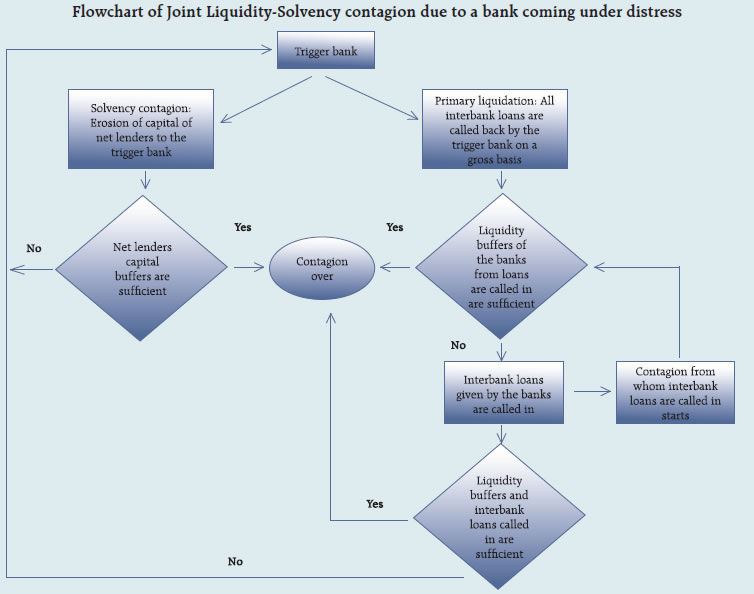The trigger bank is assumed to have failed for some endogenous reason, i.e., it becomes insolvent and thus impacts all its creditor banks. At the same time it starts to liquidate its assets to meet as much of its obligations as possible. This process of liquidation generates a liquidity contagion as the trigger bank starts to call back its loans.

The lender/creditor banks that are well capitalised will survive the shock and will generate no further contagion. On the other hand, those lender banks whose capital falls below the threshold will trigger a fresh contagion. Similarly, the borrowers whose liquidity buffers are sufficient will be able to tide over the stress without causing further contagion. But some banks may be able to address the liquidity stress only by calling in short term assets. This process of calling in short term assets will again propagate a contagion.

The contagion from both the solvency and liquidity side will stop/stabilise when the loss/shocks are fully absorbed by the system with no further failures.

1 Slippages are fresh accretion to NPAs during a period. Slippage Ratio = Fresh NPAs/Standard Advances at the beginning of the period.

Top